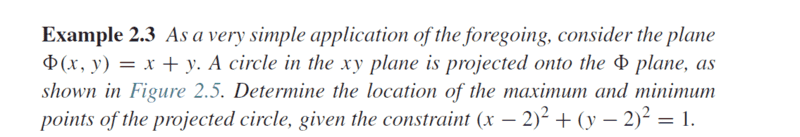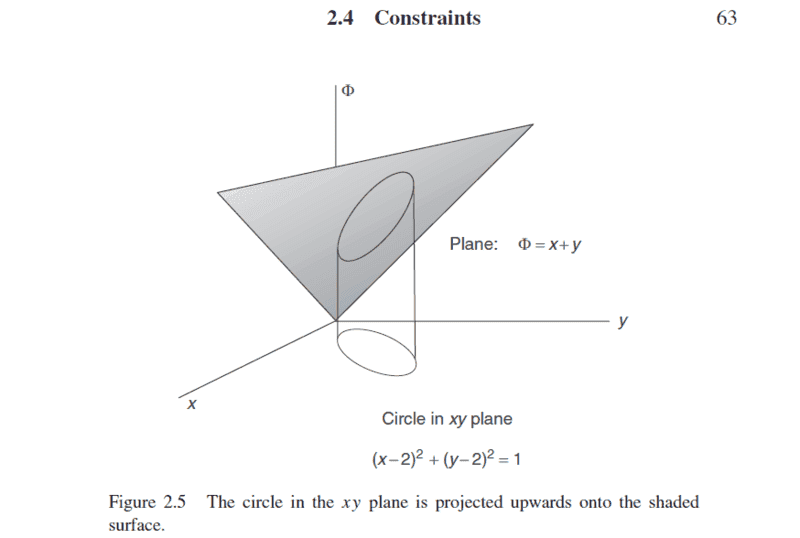# Determining maximum and minimum points of a projected circle

• Pushoam

## Homework Statement## The Attempt at a Solution

This problem belongs to the topic "calculus of variation ". The fundamental problem of “calculus of variation” is to find a function y(x) such that the integral ## I = \int_{x_i }^{ x_f} \phi (y’, y, x) ~d x ## is extremum, where ## \phi (y', y, x) ## is a functional. Then, I have to use Euler - Lagrange Equation to find out y(x).

Here, I am not able to formulate the problem using the calculus of variation technique.

Another approach is to find out the function expressing the circle in ## \phi ## - plane, i.e. f( ##\phi ## , y,x) and then use df = 0 to find out the points of maximum and minimum. Then I don’t know how to find out the function f?

Is this correct till now?

#### Attachments

I don't know what a maximum point is (or a minimum point). But if you have to find the points where ##\Phi## is at an extremum, then I doubt the need for Euler. Lagrange (actually, his multipliers method) can do it on his ownLast edited:
•Pushoam
1. Th(ue problem statement, all variables and given/known data
View attachment 226690View attachment 226695

## The Attempt at a Solution

This problem belongs to the topic "calculus of variation ". The fundamental problem of “calculus of variation” is to find a function y(x) such that the integral ## I = \int_{x_i }^{ x_f} \phi (y’, y, x) ~d x ## is extremum, where ## \phi (y', y, x) ## is a functional. Then, I have to use Euler - Lagrange Equation to find out y(x).

Here, I am not able to formulate the problem using the calculus of variation technique.

Another approach is to find out the function expressing the circle in ## \phi ## - plane, i.e. f( ##\phi ## , y,x) and then use df = 0 to find out the points of maximum and minimum. Then I don’t know how to find out the function f?

Is this correct till now?

You just have two simple constrained optimization problems:
(1) For maximum height: ##\max \, (x+y)##, subject to ##(x-2)^2 + (y-2)^2=1##.
(2) For minimum height: ##\min \, (x+y)##, subject to ##(x-2)^2 + (y-2)^2=1##.

Neither problem involves anything like "calculus of variations", although they could involve "Lagrange multipliers", depending on how you solve them.

Actually, both problems can be solved easily without calculus of any kind, just by looking at the geometry.

•Pushoam
It doesn't even require calculus. If ##x = 2+\cos\theta,~y=2 + \sin\theta##, what is ##z##? Simple trigonometry will give its maximum.

•Pushoam
Thanks for the replies. I got it.